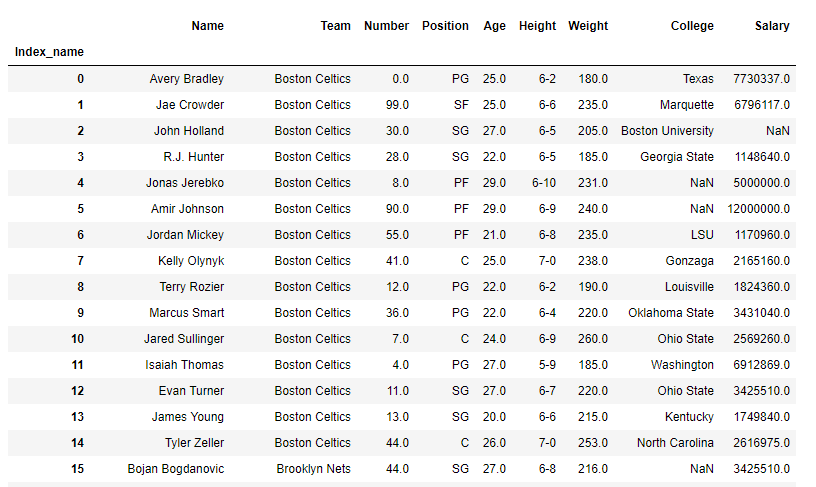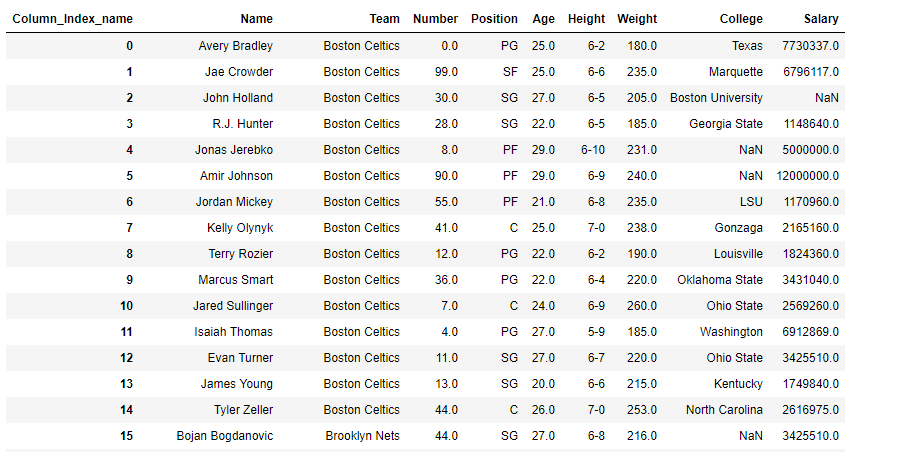Open in App
Not now

# Setting the name of the axes in Pandas DataFrame

• Last Updated : 23 Jan, 2019

There are multiple operations which can be performed over the exes in Pandas. Let’s see how to operate on row and column index with examples.

### Reset the name of the row index.

Code #1 : We can reset the name of the DataFrame index by using `df.index.name` attribute.

 `# importing pandas as pd ` `import` `pandas as pd ` ` `  `# read the csv file and create DataFrame ` `df ``=` `pd.read_csv(``'nba.csv'``) ` ` `  `# Visualize the dataframe ` `print``(df) `

Output :`# set the index name ` `df.index.name ``=` `'Index_name'` ` `  `# Print the DataFrame ` `print``(df) `

Output :Code #2 : We can reset the name of the DataFrame index by using `df.rename_axis()` function.

 `# importing pandas as pd ` `import` `pandas as pd ` ` `  `# read the csv file and create DataFrame ` `df ``=` `pd.read_csv(``'nba.csv'``) ` ` `  `# reset the index name ` `df.rename_axis(``'Index_name'``, axis ``=` `'rows'``) ` ` `  `# Print the DataFrame ` `print``(df) `

Output :### Reset the name of the column axes

Code #1 : We can reset the name of the DataFrame column axes by using `df.rename_axis()` function.

 `# importing pandas as pd ` `import` `pandas as pd ` ` `  `# read the csv file and create DataFrame ` `df ``=` `pd.read_csv(``'nba.csv'``) ` ` `  `# Visualize the dataframe ` `print``(df) `

Output :As we can see in the output, column axes of the df DataFrame does not have any name. So, we will set the name using `df.rename_axis()` function.

 `# set the name of column axes ` `df.rename_axis(``'Column_Index_name'``, axis ``=` `'columns'``) ` ` `  `# Print the DataFrame ` `print``(df) `

Output :My Personal Notes arrow_drop_up
Related Articles